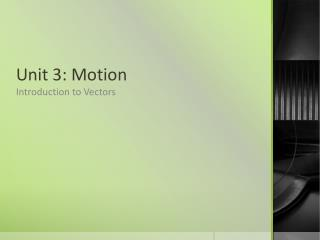Download PresentationUnit 3: MotionUnit 3: Motion - PowerPoint PPT Presentation

Download PresentationUnit 3: Motion
An Image/Link below is provided (as is) to download presentation

Download Policy: Content on the Website is provided to you AS IS for your information and personal use and may not be sold / licensed / shared on other websites without getting consent from its author. While downloading, if for some reason you are not able to download a presentation, the publisher may have deleted the file from their server.

- - - - - - - - - - - - - - - - - - - - - - - - - - - E N D - - - - - - - - - - - - - - - - - - - - - - - - - - -
Presentation Transcript

1. Unit 3: Motion Introduction to Vectors

2. B. Vectors • Scalar units of measurement that involve no direction (mass, volume, time). • Vector a physical quantity having both magnitude and direction (displacement, velocity, acceleration).

3. Distance vs Displacement • distance-- a scalar quantitythat refers to how far an object has moved during its motion. • displacement is a vector quantitythat refers to how far out of place an object is; it is the object's change in position.

4. To test your understanding of this distinction, consider the motion depicted in the diagram below. A science teacher walks 4 meters East, 2 meters South, 4 meters West, and finally 2 meters North.

5. Assignment • Distance vs Displacement (9-14)

6. Determining Angles • Before we move on to learn these methods we should learn about angles and direction

7. Angles • There are two ways to show direction • Compass direction • RCS system

8. Examples of Directions

9. Assignment • Finding Directions

10. Sample Problem A A helicopter takes off from a point A and flies due east 30km and then lands. If the helicopter takes off again and flies 40km in a straight line, how far is it from its original position? We don’t have enough information, so let’s consider the possibilities.

11. 1) If both displacements are in the same direction, the displacement would be: 30km 40km 30km + 40km = 70km east

12. 2) If displacements are in opposite directions, the helicopter would be 10km west of it’s start 30 km 40 km 30km east + 40km west = 10km west

13. 3) If the helicopter went due north after landing, the problem gets tougher. R 40 km 30 km • R represents the resultant vector. (the displacement of the helicopter) • There are 2 ways to solve this- TIP TO TAIL METHOD or VECTION ADDITION METHOD

14. 1.Vector Addition - Tail to Tip Method: • 1.) Start by placing any vector with its tail at the origin • 2.) Place the tail of another vector to the tip of the previous vector • 3.) After placing all the vectors in this fashion, the resultant vector is found by drawing a straight line from the tail of the first vector to the tip of the last vector.

15. Ex. • Given the following vectors, find the resultant vector. • ** NOTE: Order of placement (addition) does NOT matter!

16. Animation to show vector placement • http://www.physicsclassroom.com/mmedia/vectors/ao.cfm

17. Sample Problem B  A man walks 6 blocks east and then 8 blocks south. What is the resultant displacement? (Solve using the vector addition method) Step 1  choose a suitable scale. 1cm = 1 block Step 2  draw your diagram, make sure that it is accurate

18. 6 Blocks [E] 8 blocks [S] R Step 3  measure the resultant vector. In this example, R is 10cm long, therefore the displacement is 10 blocks. To find the direction, use your protractor. You should find that the displacement is 217o

19. Assignment • Tip to Tail (graphical) Vector addition

20. 2. Mathematical Vector Addition • This method will give much more accurate results • If two vectors are at right angles to each other, R can be calculated using the Pythagorean Theorem • a2 + b2 = c2

21. Sample Problem 1  A pilot is flying north at a speed of 80km/h however the wind is blowing at 40km/h from west to east. Determine the velocity of the plane mathematically. To find magnitude, we use: a2 + b2 = c2 402 + 802 = c2 1600 + 6400 = c2 8000 = c2 √8000 = √c2 c = 89.4km/h 40 km/h [E] 80 km/h [N] R θ

22. Sample Problem 2  A boat crosses a river at a velocity of 26.3 km/h [S]. If the river is flowing a velocity of 5.6 km/h [W], what is the resultant velocity of the boat? If the boat travels at this velocity for 15 minutes, what is the displacement of the boat?

23. θ a2 + b2 = c2 5.62 + 26.32 = c2 31.36 + 691.69 = c2 723.05 = c2 √723.05 = √c2 c = 26.9km/h R 26.3 km/h [S] 5.6 km/h [W]

24. Assignment • Mathematical addition of vectors